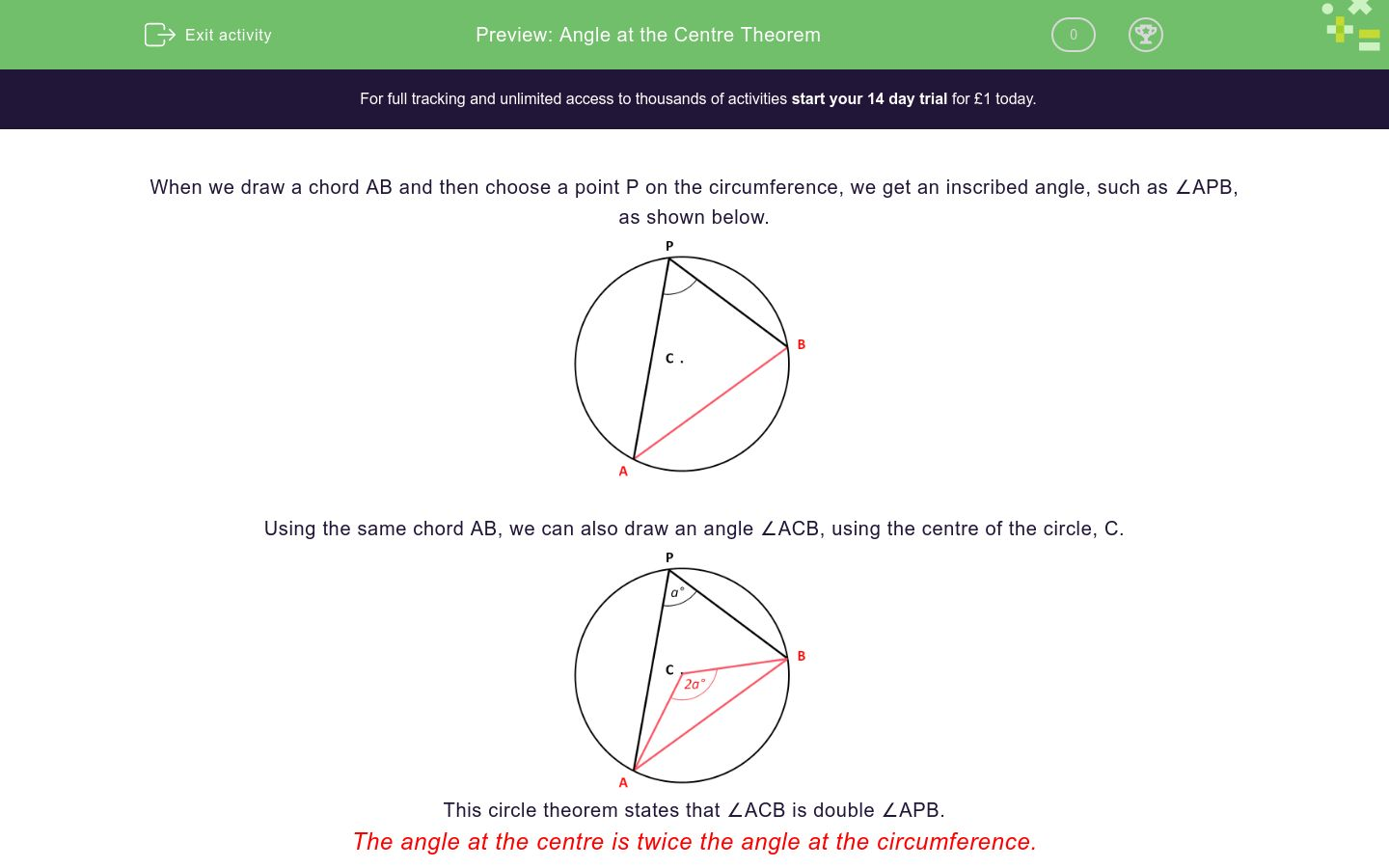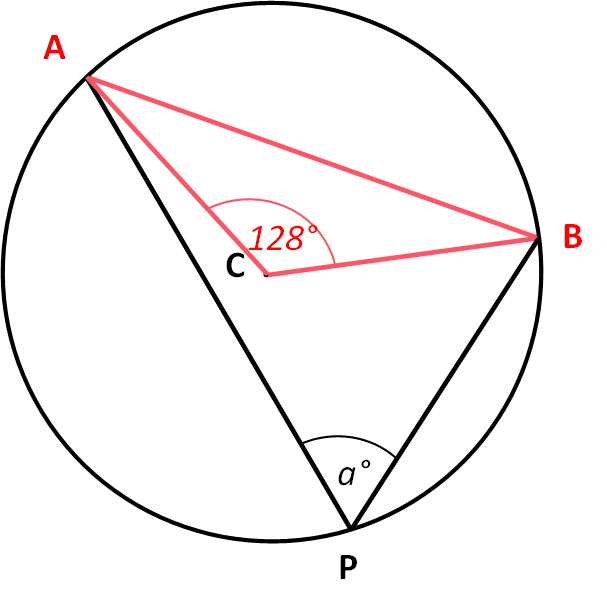# Angle at the Centre Theorem

In this worksheet, students must use the rule that an inscribed angle is half the central angle.Key stage:  KS 4

Curriculum topic:  Geometry and Measures

Curriculum subtopic:  Identify and Apply Circle Definitions and Properties

Difficulty level:### QUESTION 1 of 10

When we draw a chord AB and then choose a point P on the circumference, we get an inscribed angle, such as ∠APB, as shown below.Using the same chord AB, we can also draw an angle ∠ACB, using the centre of the circle, C.This circle theorem states that ∠ACB is double ∠APB.

The angle at the centre is twice the angle at the circumference.

Calculate the angle .

Just write the number.Calculate the angle .

Just write the number.Calculate the angle .

Just write the number.Calculate the angle .

Just write the number.Calculate the angle .

Just write the number.Calculate the angle .

Just write the number.Calculate the angle .

Just write the number.Calculate the angle .

Just write the number.Calculate the angle .

Just write the number.Calculate the angle .

Just write the number.• Question 1

Calculate the angle .

Just write the number.CORRECT ANSWER
104
EDDIE SAYS
∠ACB = 2∠APB
• Question 2

Calculate the angle .

Just write the number.CORRECT ANSWER
92
EDDIE SAYS
∠ACB = 2∠APB
• Question 3

Calculate the angle .

Just write the number.CORRECT ANSWER
73
EDDIE SAYS
∠APB = ½∠ACB
• Question 4

Calculate the angle .

Just write the number.CORRECT ANSWER
79
EDDIE SAYS
∠APB = ½∠ACB
• Question 5

Calculate the angle .

Just write the number.CORRECT ANSWER
79
EDDIE SAYS
This is similar to the previous question.
Although the position of P has moved, we still have ∠APB = ½∠ACB
• Question 6

Calculate the angle .

Just write the number.CORRECT ANSWER
78
EDDIE SAYS
∠ACB = 2∠APB
• Question 7

Calculate the angle .

Just write the number.CORRECT ANSWER
104
EDDIE SAYS
∠ACB = 2∠APB
• Question 8

Calculate the angle .

Just write the number.CORRECT ANSWER
64
EDDIE SAYS
∠APB = ½∠ACB
• Question 9

Calculate the angle .

Just write the number.CORRECT ANSWER
34
EDDIE SAYS
∠APB = ½∠ACB
• Question 10

Calculate the angle .

Just write the number.CORRECT ANSWER
74
EDDIE SAYS
∠ACB = 2∠APB
---- OR ----

Sign up for a £1 trial so you can track and measure your child's progress on this activity.

### What is EdPlace?

We're your National Curriculum aligned online education content provider helping each child succeed in English, maths and science from year 1 to GCSE. With an EdPlace account you’ll be able to track and measure progress, helping each child achieve their best. We build confidence and attainment by personalising each child’s learning at a level that suits them.

Start your £1 trial